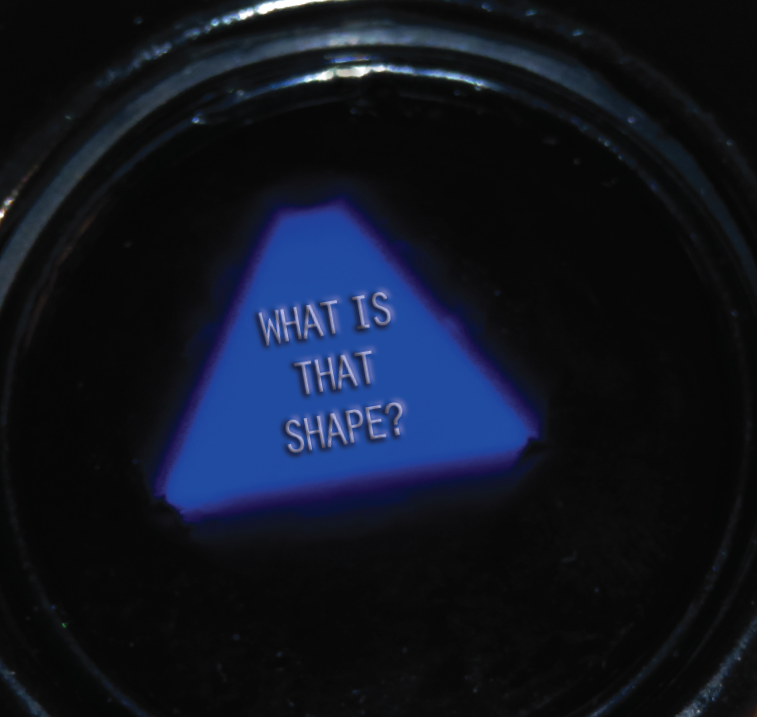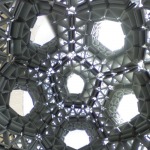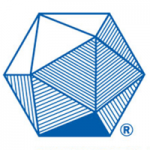# I see Platonic Solids in Your Future

#### What is the Shape Inside a Magic 8-Ball?

##### Sayonita Ghosh-Hajra

Like the MTCircular? Subscribe to our free semi-annual magazine.

What is the three-dimensional shape inside the classic Mattel Magic 8-Ball toy? The first session of the Saint Paul Elementary Math Teachers’ Circle, founded in 2017, began with this question. After a few minutes of thinking and playing, we said: CANNOT PREDICT NOW. ASK AGAIN LATER.

#### An Exploration of Platonic Solids

Platonic solids are three-dimensional closed shapes whose faces are uniform regular polygons. They have a special feature: A Platonic solid looks identical when viewed from any one of its faces. In our Circle’s first session, we explored the intriguing fact that there are only five different types of Platonic solids, whereas there are infinitely many regular polygons. Participants had fun with this hands-on activity, which relates to various geometrical concepts that can be used with third- through fifth-graders.

#### 3D Shapes from Equilateral Triangles

We began our session by reviewing regular polygons: A closed two-dimensional shape is a closed flat shape that lies on a plane, such as a circle, triangle, or square. A closed 2D shape consisting only of line segments that do not cross each other is called a polygon. A regular polygon is a polygon with all sides of equal length, and all angles of equal measure. The smallest possible regular polygon (in terms of number of sides) is an equilateral triangle, i.e., a triangle with three sides of equal length.

After reviewing regular polygons, we gave participants plastic Polydron shape tiles to play with, and we introduced the notions of face, edge, and vertex. Then we asked, “Is it possible to create a closed 3D shape such that two equilateral triangles of equal side length meet at every vertex?” SOURCES SAY NO, because the 3D shape cannot be closed.

However, participants realized that it is possible to create a closed 3D shape such that three equilateral triangles of equal side length meet at every vertex. This shape, called a tetrahedron, is an example of a Platonic solid and has three vertices, six edges, and four faces. In Greek, tetra means “four.”

Next question: “Is it possible to create a closed 3D shape such that four equilateral triangles of equal side length meet at every vertex?” OUTLOOK GOOD. This shape, an octahedron, is also a Platonic solid and has six vertices, twelve edges, and eight faces. In Greek, octa means “eight.”

“Is it possible to create a closed 3D shape such that five equilateral triangles of equal side length meet at every vertex?” WITHOUT A DOUBT! The icosahedron, a third Platonic solid, has twelve vertices, thirty edges, and twenty faces. The prefix icosa- means “twenty.”

“Is it possible to create a closed 3D shape such that six equilateral triangles of equal side length meet at every vertex?” DON’T COUNT ON IT. Participants discovered that six equilateral triangles coming together at every vertex will never be closed. We spent some time understanding why: Because each angle in an equilateral triangle measures 60 degrees, all the angles at a vertex where six equilateral triangles meet add to 360 degrees, and continuing the pattern tiles the plane.

Participants realized that when seven equilateral triangles of equal side length meet at a vertex, the sum of the angles at the vertex is more than 360 degrees, which forces the shape to have dents. Hence, there are no Platonic solids with more than five equilateral triangles meeting at every vertex.

#### Shapes from Squares

After the equilateral triangle, the next smallest regular polygon is a square. Exploring questions similar to those above, participants observed the following:

• It is not possible to create a closed 3D shape such that two squares of equal side length meet at each vertex.
• However, it is possible to form a closed 3D shape such that three squares of equal side length meet at every vertex. Such a shape is called a cube. A cube has eight vertices, twelve edges and six faces, and it is another Platonic solid.
• When four squares meet at a vertex, the sum of the angles is 360 degrees. Hence, by the same argument as for six equilateral triangles, there are no Platonic solids with more than three squares meeting at every vertex.

#### Shapes from Regular Pentagons

Next we considered regular pentagons and asked,“Is there a 3D shape such that all outer surfaces are regular pentagons of equal side length?”SIGNS POINT TO YES.

To investigate, participants examined different possibilities. They found that it is not possible to create a closed 3D shape such that two pentagons of equal side length meet at every vertex, as the shape cannot be closed.

With the manipulatives, participants found that it is possible to create a closed 3D shape with 12 regular pentagons such that three pentagons of equal side length meet at every vertex: a dodecahedron (dodeca in Greek means “twelve”). This is another Platonic solid.

Using the same logic as for equilateral triangles and squares, participants observed that if more than three pentagons meet at a vertex, the sum of the angles at that vertex will be greater than 360 degrees, meaning that there are no Platonic solids with more than three pentagons meeting at every vertex. If we consider other regular polygons with number of sides greater than 5, similar reasoning shows that it is not possible to create any other Platonic solid.

#### Conclusion

At the end of the session, we asked participants again to guess the shape inside the Magic 8-Ball. Participants recognized its faces as equilateral triangles, which indicates that it could be one of the following: a tetrahedron, an octahedron, or an icosahedron.

Once they started listing the answers given by the 8-Ball, they agreed the shape must be an icosahedron, as there are 20 possible answers. In fact, have you noticed that a number of the answers are scattered throughout this article? YES, DEFINITELY!

#### ResourcesSayonita Ghosh Hajra, a co-founder of the St. Paul Elementary Math Teachers’ Circle in Minnesota, is an Assistant Professor of Mathematics at California State University, Sacramento. The work in this article was completed during her time as an Assistant Professor at Hamline University in St. Paul.

#### MORE FROM THE FALL 2018 MTCIRCULAR#### Good Things Come in Unexpected Packages#### We the People

Can voting ever really be fair?#### The Surprising Shape of Success

Dave Honda Wins Art Exhibition Honor with Snapology Origam#### MTC Network, Initiative for Math Learning by Inquiry Receive 100Kin10 Grant#### MAA Hosts MTC Training Workshop

MAA and MTC partner to provide a workshop for teachers of underrepresented groups#### Hector Rosario Honored with Hughes Award

Co-founder of Triangle MTC recognized for outstanding contributions to county public school gifted program#### A Note from AIM

A commitment to ourselves, our students, and each other#### Dispatches from the Circles

Local updates from across the country